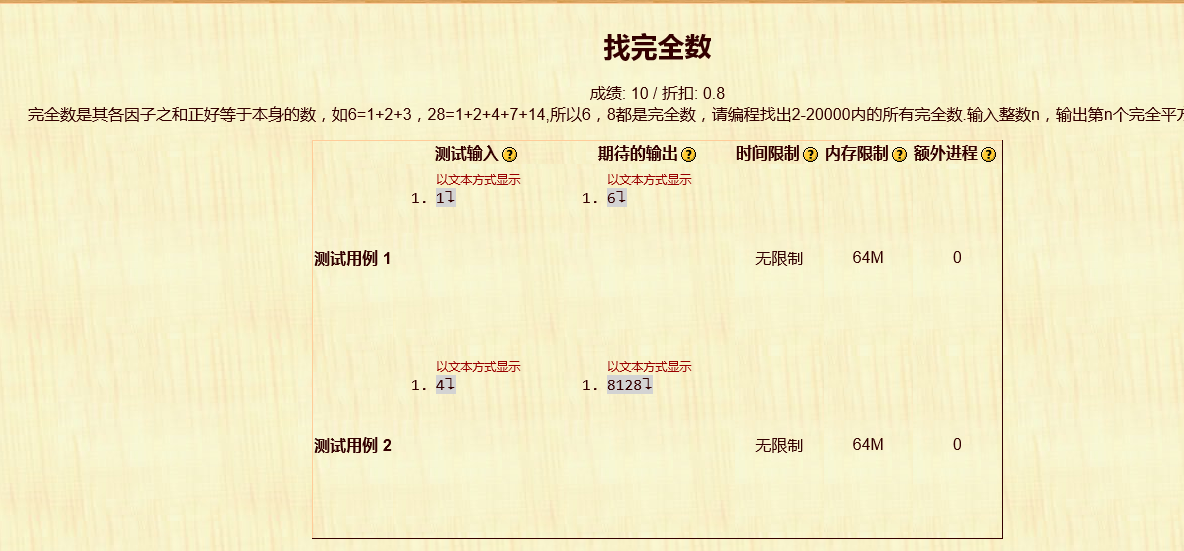c语言求完全数问题求助~~

`````` #include<stdio.h>
int main()
{
int a,i,j=0,s=0,n,b;
scanf("%d",&n);
for(a=2;a<=20000;a++)
{
for(i=1,s=0;i<a;i++)
{
if(a%i==0) s=s+i;
}

if(s==a) {b[j]=a;j++;}
}
printf("%d\n",b[--n]);
return 0;
}
``````3个回答

`````` #include<stdio.h>
#include<time.h>
int main()
{
clock_t t_s,t_e;
int a,i,j=0,s=0,n,b;
scanf("%d",&n);
t_s = clock();
for(a=2;a<=20000;a++)
{
for(i=1,s=0;i<a;i++)
{
if(a%i==0) s=s+i;
}

if(s==a) {b[j]=a;j++;}
}
t_e = clock();
double t = (t_e-t_s)/CLOCKS_PER_SEC;
printf("%d\n",b[--n]);
printf("Time used:%fs\n",t);
return 0;
}
``````

1.
20000以内只有4个完全平方数。但是事先不知道。

2.万一输入5,你的程序就出错了。要加个判断：

``````    if(n<=j) printf("%d\n",b[--n]);
else printf("未找到。");
``````

`````` for(i=1,s=0;i<=a/2;i++)
{
if(a%i==0) s=s+i;
}
``````